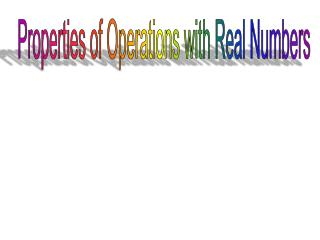# Properties of Operations with Real Numbers - PowerPoint PPT PresentationDownload PresentationProperties of Operations with Real Numbers

Properties of Operations with Real NumbersDownload Presentation## Properties of Operations with Real Numbers

- - - - - - - - - - - - - - - - - - - - - - - - - - - E N D - - - - - - - - - - - - - - - - - - - - - - - - - - -
##### Presentation Transcript

1. Properties of Operations with Real Numbers

2. Associative Property of Addition Associative Property of Addition is when the parenthesis changes in a group of numbers. Even though the parenthesis changes the problem is still the same. For example: (a+b)+c = a+(b+c) With Numbers: (1+2)+3 = 1+(2+3) Or: 6+(12+18) = (6+12)+18 Or: (22-222)+2222 = 22-(222+2222) With pictures: + + + = +

3. Associative Property of Multiplication Associative property of multiplication is when the parenthesis change in a problem. Just like the associative property of addition. For example: (ab)e = a(be) With numbers: (5x3)x2=5x(3x2) Or: (3x6)x9=3x(6x9) Or: 222x(40x5)=(222x40)x5 With pictures: = x x x x

4. Commutative Property of Addition The commutative property of addition is when order doesn’t matter. As long as you have the same numbers order doesn’t matter. For example: a+b+c = b+c+a With numbers: 5+6+8 = 6+8+5 Or: 20+55+33 = 55+20+33 Or: 232+111+445 = 111+445+232 With pictures: + + = + +

5. Commutative Property of Multiplication The commutative property of multiplication is when order doesn’t matter. Just like the commutative property of addition as long as the numbers stay the same order doesn’t matter. For example: df = fd With Numbers: 2 x 3 = 3 x 2 Or: 11 x 16 x 6 = 6 x 11 x 16 Or: 321 x 400 x 9 = 9 x 400 x 321 With pictures: x = x x x

6. Distributive Property The distributive property is when you share what’s outside of the parenthesis with what’s on the inside. This is called distributing. Usually when you distribute you are using multiplication. For example: b(c+f) = bc+bf With numbers: 5(2+6) = 5x2 + 5x6 Or: 22(11+14) = 22x11 + 22x14 Or: 453(221+112) = 453x221 + 453x112 With pictures: + + =

7. Identity Property of Addition When using the Identity property of addition you will always get the original number you started with. Always. For example: b+0=b With numbers: 4+0=4 Or: 16+0=16 Or: 636+0=636 With pictures: + =

8. Identity Property of Multiplication In the identity property of multiplication you will always get the original number. But unlike identity property of addition you usually multiply by one. For example: M(1) = M With numbers: 6x1=6 Or: 25x1=25 Or: 589x1=589 With pictures: = x

9. Multiplicative property of Zero When using the multiplicative property of zero your answer is always zero. Never changes. For example: M(0)=0 With numbers: 50 = 0 Or: 750 = 0 Or: 25030 = 0 With pictures: x =

10. Additive inverse property When using the additive inverse property the answer is always zero. It’s usually a positive and a negative number. Even when it doesn’t have the parenthesis, and the answer is still zero, it is the additive inverse property. Just like the multiplicative property of zero. For example: c + (-c) = 0 With numbers: 6+(-6) = 0 Or: -26+26=0 Or: 244-244=0 With pictures: STOP SINGING! La-la-la-la-la-la Sing a happy song. - = 0 +

11. Multiplicative Inverse Property When using the multiplicative inverse property your answer is one. Usually you are multiplying by the reciprocal. For example:a 1 a With numbers: 6 1  1 6 Or: 25 11 25 Or: 345 11 345 With pictures: =

12. Reflexive Property Any quantity is equal to itself. That means any number is equal to itself. For example: cc With numbers: 66 Or: 5555 Or: 124124 With pictures: 

13. Symmetric Property If one quantity equals another, then the second quality equals the first.These are usually if/them statements. For example: If bc then cb With numbers: If 224 then 422 Or: If 161632 then 321616 Or: If 111222333 then 333222111 With pictures:    

14. Transitive Property During the transitive property a first amount equals a second amount, and a second amount equals a third amount then the first amount equals the third. Usually an if then statement, and has three parts. For example: If mn and na then ma With numbers: If 112 and 022 then 1102 Or: If 121224 and 12224 then 1212122 Or: If 5454108 and 912108 then 5454912 With pictures:       